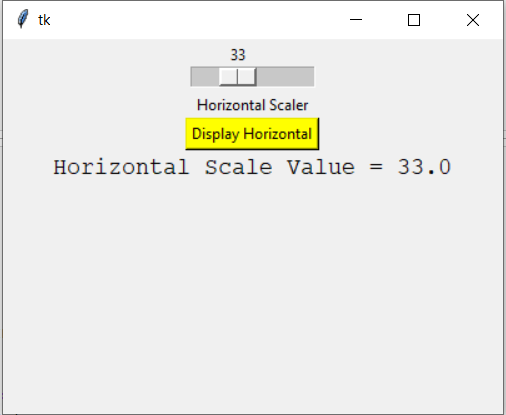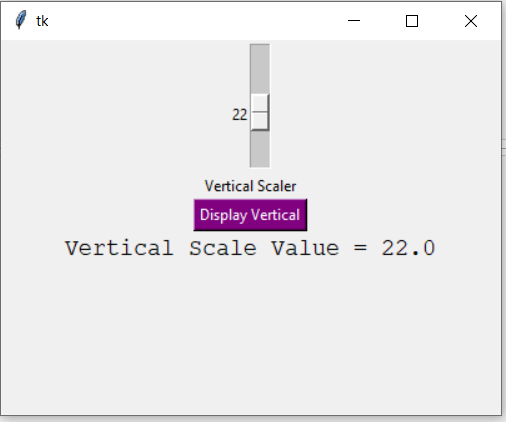# Python Tkinter – Scale Widget

Tkinter is a GUI toolkit used in python to make user-friendly GUIs.Tkinter is the most commonly used and the most basic GUI framework available in python. Tkinter uses an object-oriented approach to make GUIs.

## Scale widget

The Scale widget is used whenever we want to select a specific value from a range of values. It provides a sliding bar through which we can select the values by sliding from left to right or top to bottom depending upon the orientation of our sliding bar.

Syntax:

```S = Scale(root, bg, fg, bd, command, orient, from_, to, ..)
```

Optional parameters

• root – root window.
• bg – background colour
• fg – foreground colour
• bd – border
• orient – orientation(vertical or horizontal)
• from_ – starting value
• to – ending value
• troughcolor – set colour for trough.
• state – decides if the widget will be responsive or unresponsive.
• sliderlength – decides the length of the slider.
• label – to display label in the widget.
• highlightbackground – the colour of the focus when widget is not focused.
• cursor – The cursor on the widget ehich could be arrow, circle, dot etc.

Methods

• set(value) – set the value for scale.
• get() – get the value of scale.

Example 1: Creating a horizontal bar

 `# Python program to demonstrate ` `# scale widget ` ` `  `from` `tkinter ``import` `*`  ` `  ` `  `root ``=` `Tk()   ` `root.geometry(``"400x300"``)  ` ` `  `v1 ``=` `DoubleVar() ` ` `  `def` `show1():   ` `     `  `    ``sel ``=` `"Horizontal Scale Value = "` `+` `str``(v1.get()) ` `    ``l1.config(text ``=` `sel, font ``=``(``"Courier"``, ``14``))   ` ` `  ` `  `s1 ``=` `Scale( root, variable ``=` `v1,  ` `           ``from_ ``=` `1``, to ``=` `100``,  ` `           ``orient ``=` `HORIZONTAL)    ` ` `  `l3 ``=` `Label(root, text ``=` `"Horizontal Scaler"``) ` ` `  `b1 ``=` `Button(root, text ``=``"Display Horizontal"``,  ` `            ``command ``=` `show1,  ` `            ``bg ``=` `"yellow"``)   ` ` `  `l1 ``=` `Label(root) ` ` `  ` `  `s1.pack(anchor ``=` `CENTER)  ` `l3.pack() ` `b1.pack(anchor ``=` `CENTER) ` `l1.pack()  ` ` `  `root.mainloop() `

Output:Example 2: Creating a vertical slider

 `from` `tkinter ``import` `*` ` `  `root ``=` `Tk()   ` `root.geometry(``"400x300"``)  ` `v2 ``=` `DoubleVar() ` ` `  `def` `show2(): ` `     `  `    ``sel ``=` `"Vertical Scale Value = "` `+` `str``(v2.get())  ` `    ``l2.config(text ``=` `sel, font ``=``(``"Courier"``, ``14``)) ` ` `  `s2 ``=` `Scale( root, variable ``=` `v2, ` `           ``from_ ``=` `50``, to ``=` `1``, ` `           ``orient ``=` `VERTICAL)  ` ` `  `l4 ``=` `Label(root, text ``=` `"Vertical Scaler"``) ` ` `  `b2 ``=` `Button(root, text ``=``"Display Vertical"``, ` `            ``command ``=` `show2, ` `            ``bg ``=` `"purple"``,  ` `            ``fg ``=` `"white"``) ` ` `  `l2 ``=` `Label(root) ` ` `  `s2.pack(anchor ``=` `CENTER)  ` `l4.pack() ` `b2.pack() ` `l2.pack() ` ` `  `root.mainloop() `

Output:My Personal Notes arrow_drop_upCheck out this Author's contributed articles.

If you like GeeksforGeeks and would like to contribute, you can also write an article using contribute.geeksforgeeks.org or mail your article to contribute@geeksforgeeks.org. See your article appearing on the GeeksforGeeks main page and help other Geeks.

Please Improve this article if you find anything incorrect by clicking on the "Improve Article" button below.

Article Tags :

Be the First to upvote.

Please write to us at contribute@geeksforgeeks.org to report any issue with the above content.